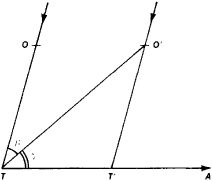# Aberration of Light

(redirected from Aberration (astronomy))

## Aberration of Light

in astronomy a change in the direction of the light ray proceeding from a celestial object, as a result of the finiteness of the speed of light and of the observer’s motion relative to the celestial body. Aberration of light causes a shift in the apparent position of the celestial body on the celestial sphere.

As the observer is displaced together with the instrument sensing the light—for example, an astronomical telescope— a certain small time interval t is required for the ray of light incident upon the objective at point O (see Figure 1) to traverse the distance from the objective O to the cross hairs T located in the focal plane of the objective. During that time the instrument experiences translational motion in the direction toward the point A (apex) and occupies the position O’ T’, while the image of the celestial body is displacedFigure 1

relative to the cross hairs in the direction opposed to the motion of the observer. To get the image of the celestial body on the cross hairs, the telescope has to be turned in the direction of motion of the observer through an angle OTO’ = β, with β expressed in seconds of arc as in the formula β = (206,264.8” v/c) sin γ where v is the velocity of the observer, c is the speed of light, and y is the angle between the directions pointing to the celestial body and to the apex. Aberration of light shifts the celestial body on the celestial sphere toward the apex, and this shift is increased with increasing velocity of the observer.

The aberrations of light are characterized as diurnal, caused by the rotation of the earth about its axis; annual, caused by the earth’s motion around the sun; and secular, the consequence of the motion of the solar system through space. Diurnal aberration of light shifts the celestial body toward the east by an amount β = 0.319” cos ϕ sin γ where ϕ is the geographical latitude of the observer. The annual aberration of light shifts the celestial body toward the instantaneous apex of the earth’s motion around the sun, so that the star describes a small ellipse on the celestial sphere in the course of one year. The annual aberration of light is β = k sin γ. The coefficient k, known as the constant of aberration, has a value of 20.496”, a value adopted in 1964 by the International Astronomical Union (the earlier value was 20.47”). The annual aberration of light was discovered and explained by J. Bradley in 1728. In the secular aberration of light, the velocity at which the observer moves is the velocity of the sun’s motion relative to the stars, which undergoes virtually no change either in numerical value or direction, so that changes introduced by that aberration into the coordinates of each star are constant and need not be taken into account.

In the derivation of the formula for aberration of light in the special theory of relativity, we consider the difference in the direction of the light ray in different inertial systems of reference. The formula for the aberration of light is derived as a natural consequence of the Lorentz transformations and in the first approximation leads to the same result as in the classical theory of aberration of light. The resultant correction to the value of the annual aberration of light does not exceed 0.0005”, and this is small enough to be ignored.

### REFERENCES

Blazhko, S. N. Kurs sfericheskoi astronomii, 2nd ed. Moscow, 1954.
Kulikov, K. A. Fundamental’nye postoiannye astronomii. Moscow, 1956.

K. A. KULIKOV

Site: Follow: Share:
Open / Close